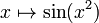# Sine of square function

This article is about a particular function from a subset of the real numbers to the real numbers. Information about the function, including its domain, range, and key data relating to graphing, differentiation, and integration, is presented in the article.
View a complete list of particular functions on this wiki
For functions involving angles (trigonometric functions, inverse trigonometric functions, etc.) we follow the convention that all angles are measured in radians. Thus, for instance, the angle of$90\,^\circ$ is measured as$\pi/2$.

## Definition

This function is defined as the composite of the sine function and the square function. Explicitly, it is the function:$x \mapsto \sin(x^2)$

Note that this is different from$\sin^2$, the sine-squared function. It also differs from the sine of sine function, obtained by composing the sine function with itself.

## Graph

Here is a zoomed out graph for the domain restricted to$[-5\sqrt{\pi},5\sqrt{\pi}]$:

## Differentiation

### Using the chain rule for differentiation

WHAT WE USE: chain rule for differentiation, differentiation rule for power functions, sine function#Differentiation

We use the chain rule for differentiation viewing the function as the composite of the square function on the inside and the sine function on the outside:$\frac{d}{dx}[\sin(x^2)] = \frac{d(\sin(x^2))}{d(x^2)} \frac{d(x^2)}{dx} = (\cos(x^2))(2x) = 2x\cos(x^2)$